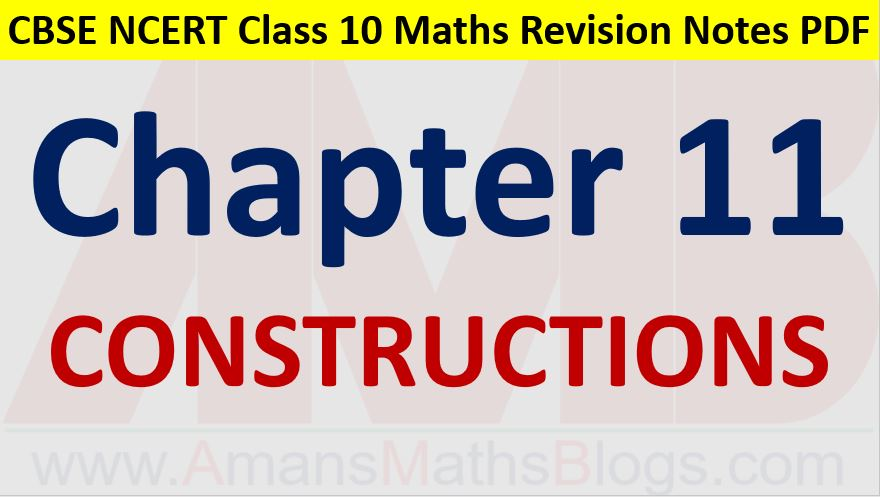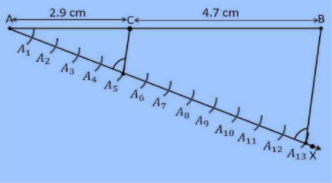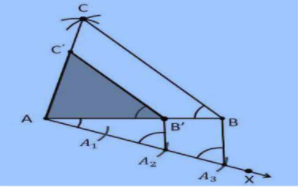Friday, October 22, 2021
Home > CBSE Class 10 > Constructions CBSE NCERT Notes Class 10 Maths Chapter 11 PDF

# Constructions CBSE NCERT Notes Class 10 Maths Chapter 11 PDFHi students, Welcome to AMBiPi (Amans Maths Blogs). In this article, you will get Constructions CBSE NCERT Notes Class 10 Maths Chapter 11 PDF. You can download this PDF and save it in your mobile device or laptop etc.

# Constructions CBSE NCERT Notes Class 10 Maths Chapter 11 PDF

## Constructions 1 : Division of a Line Segment

In the division of a line segment, a line is divided into a ratio (let, m : n).

### Class 10 Maths Chapter 11 Examples 1:

Draw a line segment of length 7.6 cm and divide it in the ratio 5 : 8. Measure the two parts.

Step of Construction :

Step 1 : Draw a line segment AB = 7.6 cm.

Step 2 : Draw a ray AX that makes an acute angle with line segment AB.

Step 3 : Locate 13 (= 5 + 8) points A1, A2, A3, …, A13 on the line on AX such that AA1 = A1A2 = … = A12A13

Step 4 : Join BA13.

Step 5 : Through the point A5 (m = 5), draw a line parallel to A13B (by making an angle equal to ∠AA13B) at A5 intersecting AB at the point C.

Step 6 : C is a point which divide AB into 5 : 8, it means AC : CB = 5 : 8. Now, measure the lengths of AC and CB. Thus, we get AC = 2.9 cm and CB = 4.7 cm.## Constructions 2 : Construction of Similar Triangle

In this, a triangle is constructed and then another triangle similar to given triangle is constructed.

### Class 10 Maths Chapter 11 Examples 2:

Construct a triangle of sides 4 cm, 5 cm and 6 cm and then a triangle similar to it whose sides are 2/3 of the corresponding sides of the first triangle.

Step of Construction :

Step 1 : Draw a line segment AB = 4 cm.

Step 2 : Take the point A as centre, and draw an arc of radius 5 cm.

Step 3 : Similarly, take the point B as centre, and draw an arc of radius 6 cm.

Step 4 : These two arcs will intersect each other at a point. Mark this point as C. This is the vertex C of the triangle ABC.

Step 5 : Now, the triangle ABC is constructed.

Step 6 : Draw a ray AX which makes an acute angle with the line segment AB in the opposite side of the vertex C.

Step 7 : Locate 3 points on AC such that AA1 = A1A2 = A2A3.

Step 8 : Join the point BA3 and draw a line thorough A2 which is parallel to the line BA3 that intersect AB at point B.

Step 9 : Through the point B’, draw a line parallel to the line BC that intersect the line AC at C.

Step 10 : Therefore, the triangle AB’C’ is the triangle, which is similar to the triangle ABC.## Constructions 3 : Construction of Tangents to a Circle

To draw a tangent at a point of a circle, simply draw the radius through this point and draw a line perpendicular to this radius through this point and this will be the required tangent at the point.

If the point lies outside the circle, there will be two tangents to the circle from this point.

### Class 10 Maths Chapter 11 Examples 3:

Draw a circle of radius 6 cm. From a point 10 cm away from its centre, construct the pair of tangents to the circle and measure their lengths.

Step of Construction :

Step 1 : Draw a circle with radius 6 cm with center O.

Step 2 : Locate the point P, which is 10 cm away from O.

Step 3 : Join the points O and P through line.

Step 4 : Draw the perpendicular bisector of the line OP.

Step 5 : Let M be the mid-point of the line PO.

Step 6 : Take M as center and measure the length MO.

Step 7 : The length MO is taken as radius and drawn another circle.

Step 8 : The circle drawn with the radius of MO, intersect the previous circle at the point Q and R.

Step 9 : Join PQ and PR.

Step 10 : Therefore, PQ and PR are the required tangents to the given circle.Click below to get CBSE Class 10 Maths Chapter wise Revision Notes PDF.

Chapter NoChapter name
Chapter 1 :Real Numbers CBSE Class 10 Maths Revision Notes
Chapter 2 :Polynomials CBSE Class 10 Maths Revision Notes
Chapter 3 :Pair of Linear Equations in Two Variables CBSE Class 10 Maths Revision Notes
Chapter 4 :Quadratic Equations CBSE Class 10 Maths Revision Notes
Chapter 5 :Arithmetic Progression CBSE Class 10 Maths Revision Notes
Chapter 6 :Triangles CBSE Class 10 Maths Revision Notes
Chapter 7 :Coordinate Geometry CBSE Class 10 Maths Revision Notes
Chapter 8 :Introduction to Trigonometry CBSE Class 10 Maths Revision Notes
Chapter 9 :Some Applications of Trigonometry CBSE Class 10 Maths Revision Notes
Chapter 10 :Circles CBSE Class 10 Maths Revision Notes
Chapter 11 :Constructions CBSE Class 10 Maths Revision Notes
Chapter 12 :Area Related to Circles CBSE Class 10 Maths Revision Notes
Chapter 13 :Surface Areas & Volumes CBSE Class 10 Maths Revision Notes
Chapter 14 :Statistics CBSE Class 10 Maths Revision Notes
Chapter 15 :Probability CBSE Class 10 Maths Revision Notes
AMBiPi
error: Content is protected !!